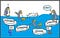# Hyper Parameter Tuning Using Grid search and Random search

A hyperparameter is a parameter that controls the learning process of the machine learning algorithm. Hyperparameter Tuning is choosing the best set of hyperparameters that gives the maximum performance for the learning model.

# Model Parameters

In a machine learning model, training data is used to learn the weights of the model. These weights are the Model parameters.

Example: In a linear regression model, the model is trained to produce the accurate prediction of the function y=mx + b, where m is slope and b is intercept.

Here m and b are the model parameters whose best values are chosen by training the…

# HDF5 file format with Pandas

HDF5 is a data format that stores and manages large and complex data with lesser disk space and faster retrieve.

While reading and working on large datasets, we often run into out of memory error because of the large memory used. Storing data is some other format which is fast to access and smaller in size, is one of the solutions for this problem. One such file format is HDF5.

HDF stands for Hierarchical Data Format. Most common version used is Version 5. A HDF file can store any kind of heterogeneous data objects such as images, arrays, tables, graphs…

# Feature Scaling

Feature Scaling is a method to transform the numeric features in a dataset to a standard range so that the performance of the machine learning algorithm improves. It can be achieved by normalizing or standardizing the data values. This scaling is generally preformed in the data pre-processing step when working with machine learning algorithm.

Example, if we have weight of a person in a dataset with values in the range 15kg to 100kg, then feature scaling transforms all the values to the range 0 to 1 where 0 represents lowest weight and 1 represents highest weight instead of representing the…

# Gradient Boost for Regression Explained

Gradient boost is a machine learning algorithm which works on the ensemble technique called ‘Boosting’. Like other boosting models, Gradient boost sequentially combines many weak learners to form a strong learner. Typically Gradient boost uses decision trees as weak learners.

Gradient boost is one of the most powerful techniques for building predictive models for both classification and Regression problems. In this blog we will see how Gradient boost works with Regression.image Talking about Thinking

Boosting?

Boosting idea is to train weak learners sequentially, each trying to correct its predecessor. This means, the algorithm is always going to learn something which is not completely…

# Regularization in Linear Models

In machine learning, Regularization is a modification to be applied on a model so that we get more generalized model that fits well with new data.

Linear regression is an approach to find the relationship between variables using a straight line. It tries to find a line that best fits the data.

# Understanding AdaBoost

AdaBoost is adaptive learning algorithm, which learns from calling weak learning algorithms repeatedly in a sequential way. It adapts to the error rates of individual weak hypothesis. This is the basis of its name- “ada” means adaptive. It is an ensembled boosting model.

First let us see what is boosting and then understand how AdaBoost works.

# Boosting

Boosting is a technique of combining set of weak learners into a strong learner.

A weak learner is a classifier whose performance is poor (accuracy is slightly better than a random guess). In contrast, a strong learner is a classifier with arbitrarily high accuracy.

# Decision tree vs SVM

A Decision tree is a tool in Machine learning that is tree like model which uses some conditions to arrive at a consequence. Each Condition is a If-Else like statement (Example When coin flipped, If heads Else Tails).

Decision tree works with limited amount of data and when features are non-monotonic. A small change in data changes the tree structure. It works will with structured data. It requires less training time. Performance is less comparatively. Due to tree like structure, it is easy to understand but difficult to scale to large dataset. …## Ravali Munagala

Data Scientist & Machine Learning Engineer